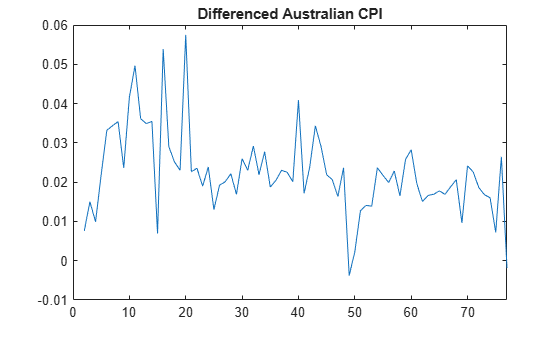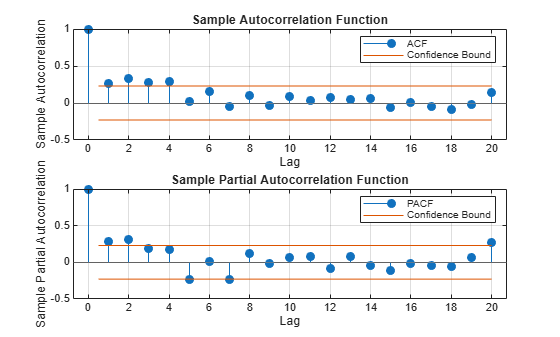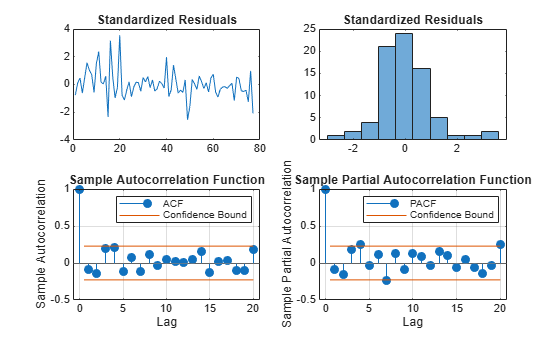Main Content

# Infer Residuals for Diagnostic Checking

This example shows how to infer residuals from a fitted ARIMA model. Diagnostic checks are performed on the residuals to assess model fit.

The time series is the log quarterly Australian Consumer Price Index (CPI) measured from 1972 to 1991.

### Load the Data.

Load the Australian CPI data. Take first differences, then plot the series.

```load Data_JAustralian y = DataTable.PAU; T = length(y); dY = diff(y); figure plot(2:T,dY) xlim([0,T]) title('Differenced Australian CPI')```The differenced series looks relatively stationary.

### Plot the Sample ACF and PACF.

Plot the sample autocorrelation function (ACF) and partial autocorrelation function (PACF) to look for autocorrelation in the differenced series.

```figure subplot(2,1,1) autocorr(dY) subplot(2,1,2) parcorr(dY)```The sample ACF decays more slowly than the sample PACF. The latter cuts off after lag 2. This, along with the first-degree differencing, suggests an ARIMA(2,1,0) model.

### Estimate an ARIMA(2,1,0) Model.

Specify, and then estimate, an ARIMA(2,1,0) model. Infer the residuals for diagnostic checking.

```Mdl = arima(2,1,0); EstMdl = estimate(Mdl,y);```
``` ARIMA(2,1,0) Model (Gaussian Distribution): Value StandardError TStatistic PValue __________ _____________ __________ __________ Constant 0.010072 0.0032802 3.0707 0.0021356 AR{1} 0.21206 0.095428 2.2222 0.02627 AR{2} 0.33728 0.10378 3.2499 0.0011543 Variance 9.2302e-05 1.1112e-05 8.3066 9.8491e-17 ```
`[res,~,logL] = infer(EstMdl,y);`

Notice that the model is fit to the original series, and not the differenced series. The model to be fit, `Mdl`, has property `D` equal to `1`. This accounts for the one degree of differencing.

This specification assumes a Gaussian innovation distribution. `infer` returns the value of the loglikelihood objective function (`logL`) along with the residuals (`res`).

### Perform Residual Diagnostic Checks.

Standardize the inferred residuals, and check for normality and any unexplained autocorrelation.

```stdr = res/sqrt(EstMdl.Variance); figure subplot(2,2,1) plot(stdr) title('Standardized Residuals') subplot(2,2,2) histogram(stdr,10) title('Standardized Residuals') subplot(2,2,3) autocorr(stdr) subplot(2,2,4) parcorr(stdr)```The residuals appear uncorrelated and approximately normally distributed. There is some indication that there is an excess of large residuals.

### Modify the Innovation Distribution.

To explore possible excess kurtosis in the innovation process, fit an ARIMA(2,1,0) model with a Student's t distribution to the original series. Return the value of the loglikelihood objective function so you can use the Bayesian information criterion (BIC) to compare the fit of the two models.

```MdlT = Mdl; MdlT.Distribution = 't'; [EstMdlT,~,logLT] = estimate(MdlT,y);```
``` ARIMA(2,1,0) Model (t Distribution): Value StandardError TStatistic PValue _________ _____________ __________ __________ Constant 0.0099745 0.0016152 6.1753 6.6058e-10 AR{1} 0.32689 0.075503 4.3294 1.495e-05 AR{2} 0.18719 0.074691 2.5063 0.012202 DoF 2.2594 0.95562 2.3643 0.018064 Variance 0.0002472 0.00074618 0.33129 0.74043 ```
`[~,bic] = aicbic([logLT,logL],[5,4],T)`
```bic = 1×2 -492.5317 -479.4691 ```

The models with the t-innovation distribution (`MdlT` and `EstMdlT`) have one extra parameter (the degrees of freedom of the t distribution).

According to the BIC, the ARIMA(2,1,0) model with a Student's t innovation distribution is the better choice because it has a smaller (more negative) BIC value.

Download ebook